#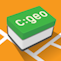c:geo User Guide

### Sidebar

en:coordinatedialog

# Coordinate input dialog

The coordinate input dialog is opened if you click on the coordinate field while editing a waypoint or for entering coordinates in the search or Go to menus.

Thebutton will close the dialog without changes, thewill close the dialog and save the changes.

Be sure to save the changes especially when using the waypoint calculator as no valid coordinates might be shown, but you still want to save the recent changes you made in the calculator.

## Coordinate formatBy clicking on this field you can select between the different supported coordinate formats or selecting `Plain` to manually input the desired format. The coordinate input fields will change their layout depending on your selection here.

If you already entered coordinates these will be converted into the selected format.

## Coordinate input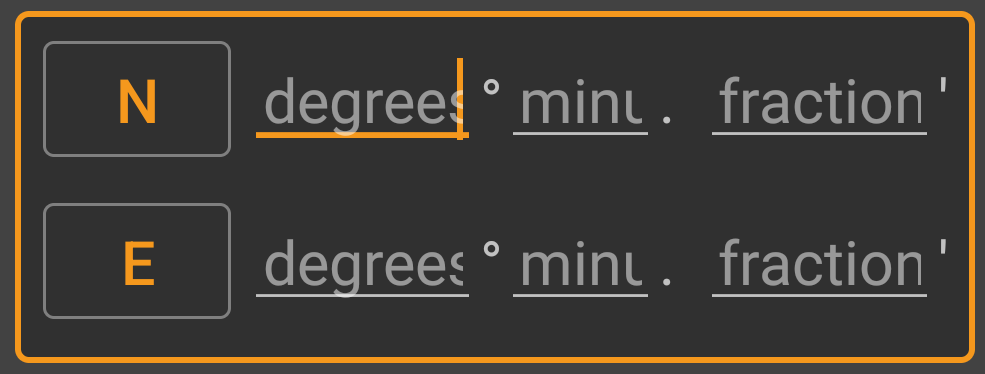Use these fields to enter the coordinates. By clicking on N/W you can change to S/E as needed.

The layout of these input field depends on the coordinate format you selected above.

## Coordinate sources

Below the coordinate input fields you will find several buttons allowing to insert coordinates from different sources. All or a subset of these buttons might be shown depending on where you use the coordinate input dialog and other conditions.

Button Description
My coordinatesWill insert your current position into the coordinate fields.
Cache coordinatesWill insert the cache listing coordinates into the coordinate fields.
Calculate coordinatesWill use the coordinates already entered above (optionally) and open the waypoint calculator.
From clipboardThis button is only shown if your clipboard contains valid coordinates and will paste them into the coordinate fields.
Clear coordinatesEmpties the coordinate fields.

## Waypoint Calculator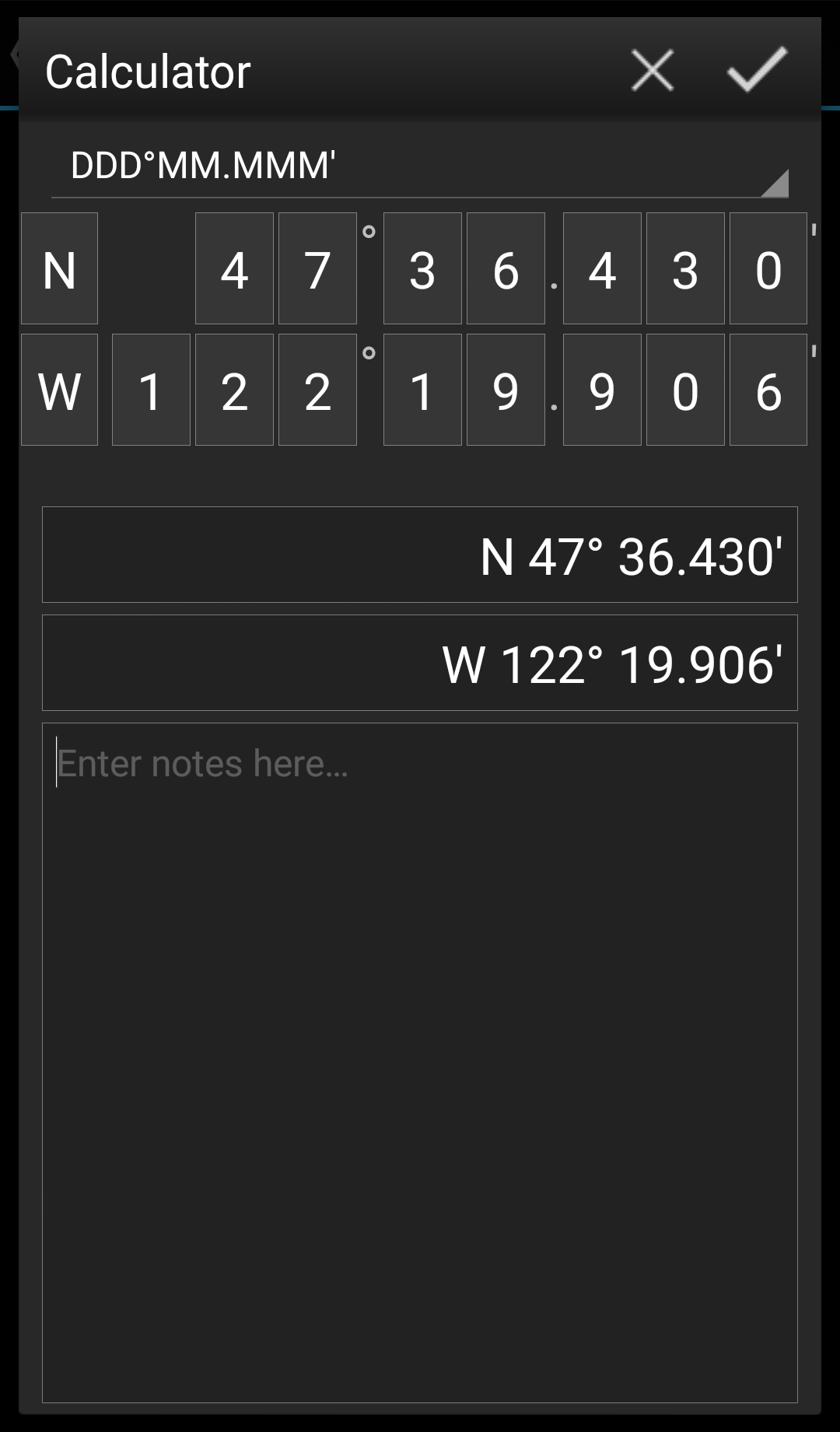c:geo offers a calculator for waypoints, which allows to make use of variables and formulas to calculate coordinates based on the result.

Once you clicked on `Calculate Coordinates` in the coordinate input dialog this screen will be shown.

Thebutton will close the dialog without changes, thewill close the dialog, save the changes and return to the waypoint editor.

You can use the calculator also for preparing your field trip by entering the needed variables and formulas but not yet entering values for your variables.
This will of course not yet provide a valid result, but you can still save the current state at any time by saving your changes in the calculator and afterwards saving the waypoint state.

Clicking on the first field will open a drop down menu allowing to change between different coordinate formats as explained here. All further functions are explained below.

For easier understanding we will use an example of how the calculator can be used.

### Main variable assignment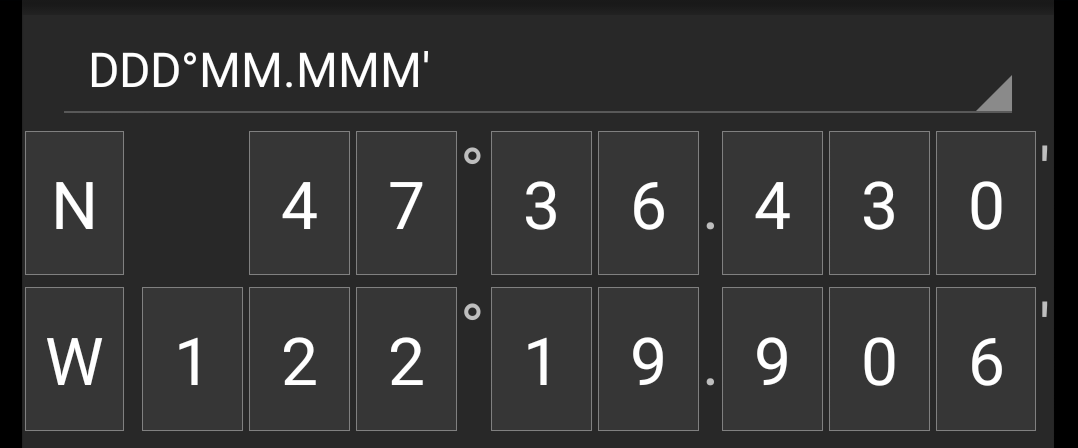By default this section will show the cache coordinates as a starting point for assigning your variables.

By short clicking on each digit you can cycle between the following functions for this specific digit:

• Assigning a variable to this digit
Will assign the next unused variable (A-Z) to this digit.
• Omit this digit
Will remove this digit completely from the calculation. This will allow solutions with more than one digit in the neighboring variables.
Will show the original value again.

Furthermore you can long click each digit to manually enter a number or variable.

The result could look like this example: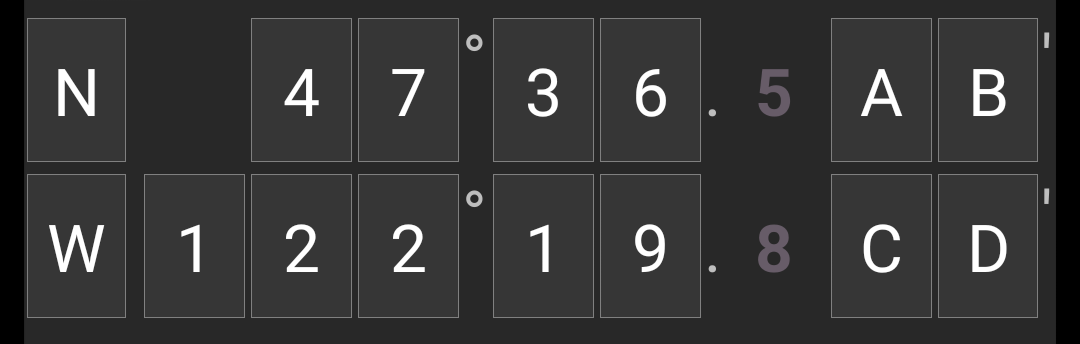### Main variable input

For the main variables you always have to use CAPITAL letters (A-Z), whereas for the sub variables (described further down) you always have to use lower case letters (a-z)!

The section below the coordinate field will show fields for all the main variables you assigned in the coordinate field. In our example it will look like this as we assigned four main variables (A,B,C,D):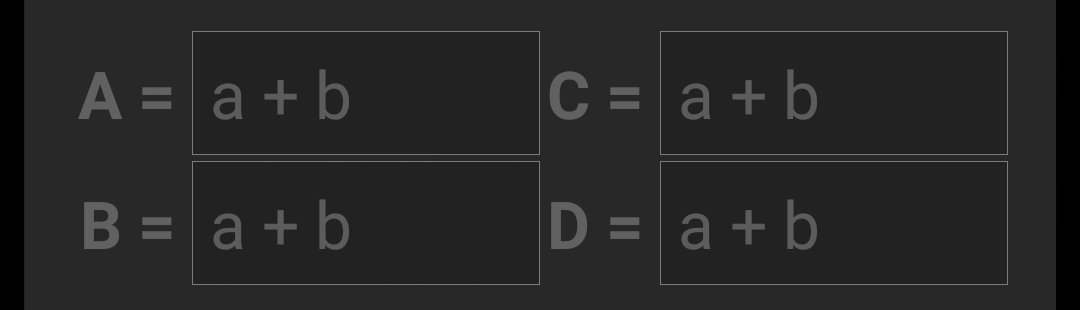You can now use these fields to assign a formula using sub variables (lower case a-z) to calculate the result for the main variables.

The following operators are supported in these formulas:

Operator Function Example
-Subtractiona-b
*Multiplicationa*b
/Divisiona/b
^Potentiatea^b
%Moduloa%10
( )Parenthesis(a+b)*c

In our example, we use the following formulas for the main variables (as e.g. provided by the cache owner in the cache description) to continue: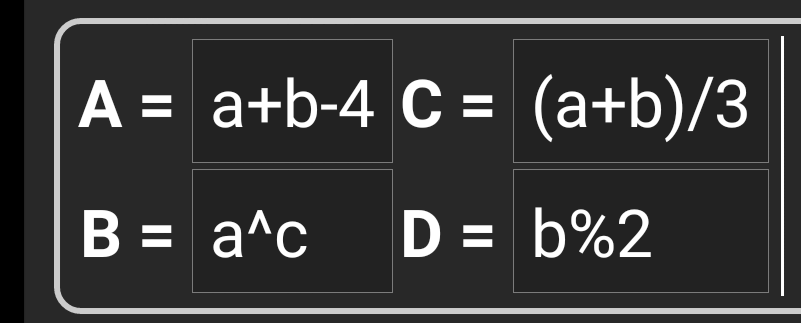### Sub variable assignment

For the sub variables you always have to use lower case letters (a-z), whereas for the main variables (described further above) you always have to use CAPITAL letters (A-Z)!

After assigning formulas to the main variables, an input field will be added automatically for each sub variable (lower case a-z) used in these formulas.

These input fields can now be used to enter numeric values, which will then be used for automatic calculation of the result coordinates using the formulas provided. You can close and save the calculator state at any time now and return to the calculator to enter the next sub variable value as soon as you found it while doing your cache.

Depending on the amount of main and sub variables you have to swipe the variable section to reach all input fields.
You might also rotate your device into landscape mode to have all variables visible on your display.

In our example we did now find the values for a,b and c and entered them accordingly: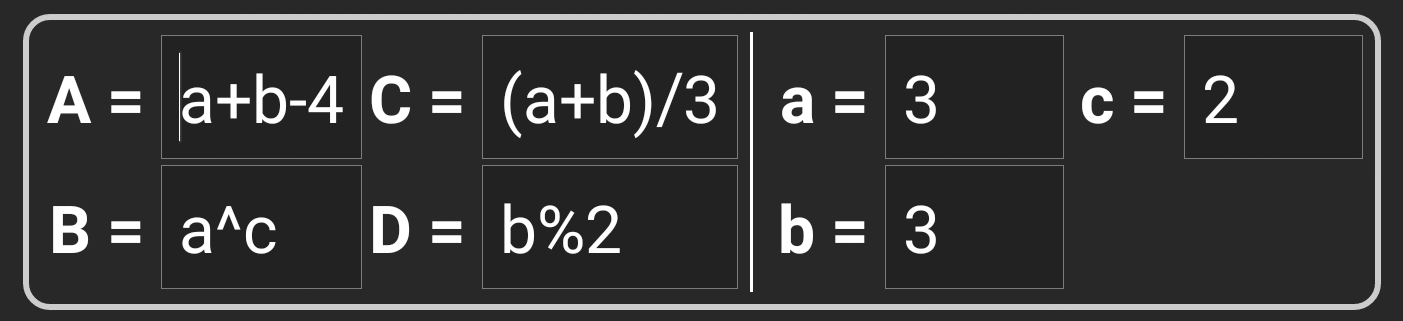### Result prediction

While you enter the formulas for the main variables and the values for the sub variables the result will be shown - on the fly in the result section.

Digits not yet valid will be replaced by `*` and greyed out. Once the result produces valid coordinates the result field will be shown with all white characters.

In our example a valid result is shown: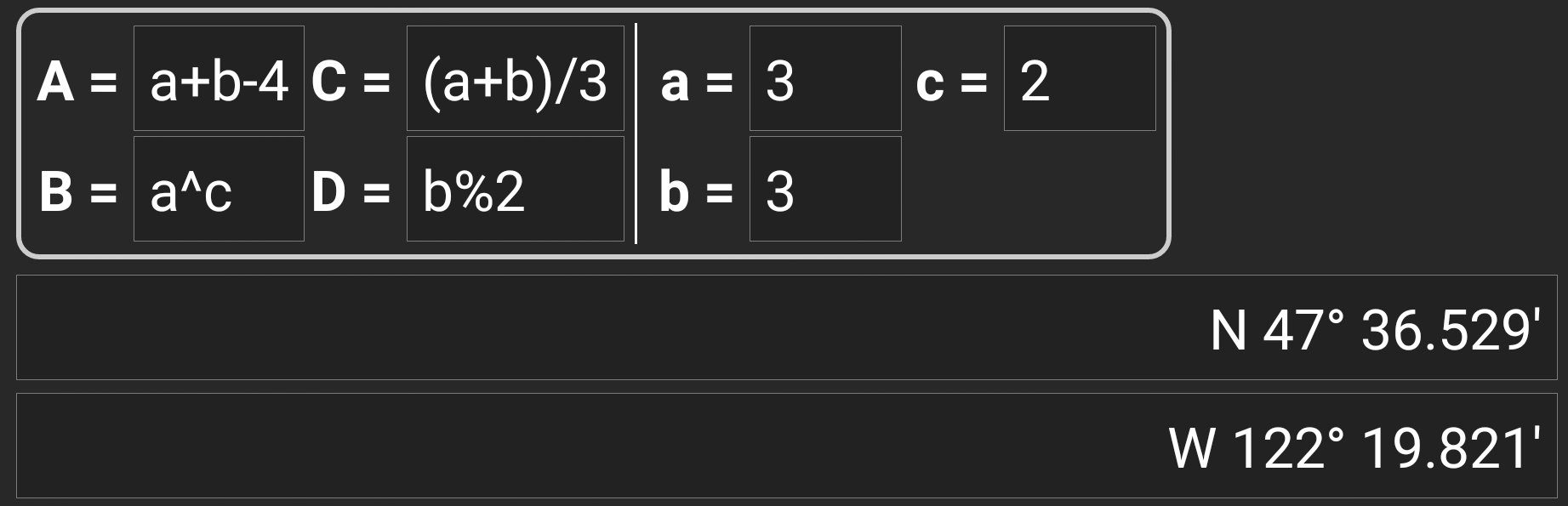### Calculator notes

The last section in the calculator can be used to enter or paste notes, which can be helpful to e.g. copy parts of the cache description containing the calculations required to find the cache.

The content of the calculator note will be synchronized to the note of the waypoint used to calculate.

### Using Plain Coordinate Format

Entering coordinates or coordinate formulas in Plain mode works completely different compared to the fixed formats. Instead of having a clickable field for each digit there are two free text fields to enter the latitude and longitude part of a coordinate.

A single digit or a group of digits can be replaced by a formula surrounded with parenthesis. The variables used in the formulas must consist oft single CAPITAL letters.

Often cache listings provide their waypoint coordinates already in this expected format. Therefore the plain mode is best suited when coordinates should be taken over “as is” from the listings.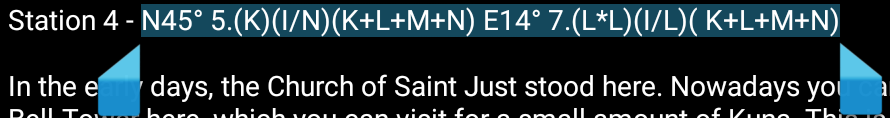The Plain mode works best with coordinates in MinDeg format `DDD° MM.MMM`'.

To enter the Plain mode change the “Coordinate format” to `Plain`.

Taking the example in the screenshot above, copy the latitude (N/S) part of the coordinate into the first input field and the longitude part (E/W) into the second. If the coordinate contains formulas with variables the corresponding variable input fields appear below.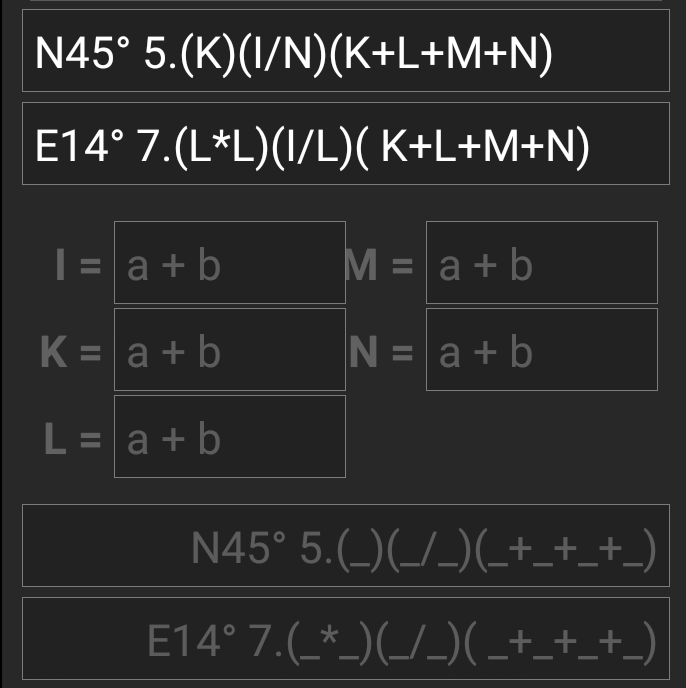The formulas from the screenshot produce input fields for I, K, L, M and N. You can fill the variable fields with values in the same way as with the fixed formats described in the previous sections.

As soon as the input for all variable fields is complete the result prediction usually turns into white and shows the calculated coordinate.In some cases the result field still stays gray because the coordinate parser could not interpret the initial coordinate input correctly. In the screenshot below there is a misplaced SPACE character between two digits of the same group which confuses the coordinate parser.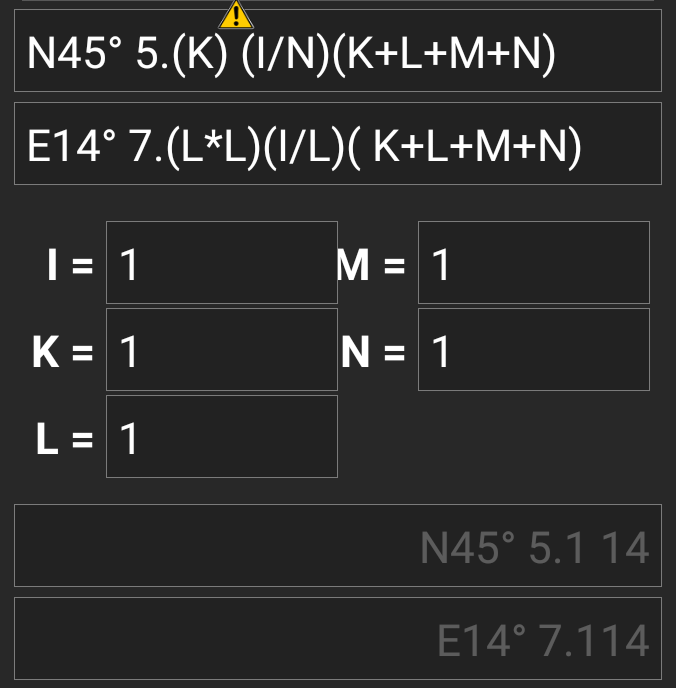Typical problems with coordinates copied from listings are:

• Lower case variable names; variables must consist of capital letters
• Space between digits within a group `N45° 5.(K) (I/N)(K+L+M+N)`
• Space before the degree sign `N45 ° 5.(K)(I/N)(K+L+M+N)`
• Space before the decimal point `N45° 5 .(K)(I/N)(K+L+M+N)`
• Space after the decimal point `N45° 5. (K)(I/N)(K+L+M+N)`
• Wrong minus sign: There are many different dash signs - only the standard hyphen/minus sign (`-`) is accepted
• Wrong multiplication sign: sometimes an `x` or an interpunct (`·`) is taken as multiplier sign instead of an asterisk (`*`)
• Wrong division sign: sometimes a colon (`:`) is taken as division sign instead of a slash (`/`)
• Nested square brackets: `N45° 5.[[X+Y]*10]`

As with the other formats above the state of calculator can be saved at any time.

### Further information

The contributor of the calculator code, published some videos presenting different usage examples for the calculator: